S T E E R ,   A N D   R E L A Y   V A L V E   B O D Y   A S S E M B L I E S   - R E P A I R    A N D    R E B U I L D    S T A N D A R DS
Custom Search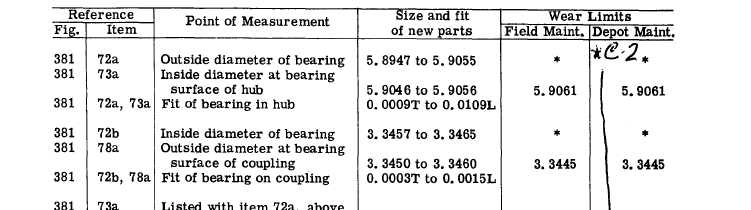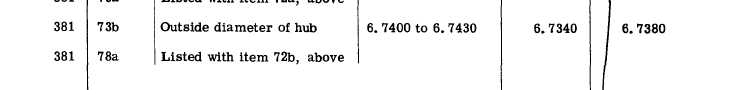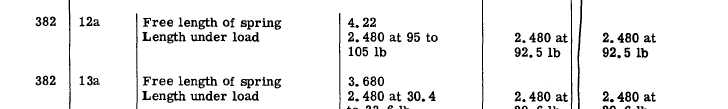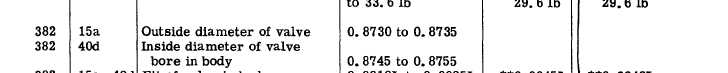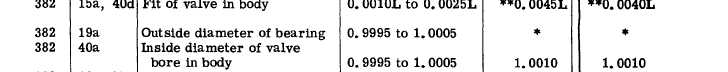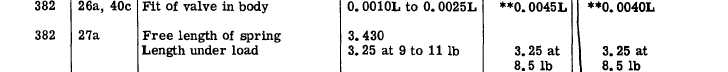*C2 P A R   2 4 4 - 2 4 5 R E P A I R     A N D     R E B U I L D     S T A N D A R DS C  H  A  P    6 2 4 5 .    S T E E R ,    A N D    R E L A Y    V A L V E    B O D Y    A S S E M B L I E S    - R E P A I R     A N D     R E B U I L D     S T A N D A R DS 221Integrated Publishing, Inc. - A (SDVOSB) Service Disabled Veteran Owned Small Business